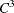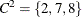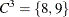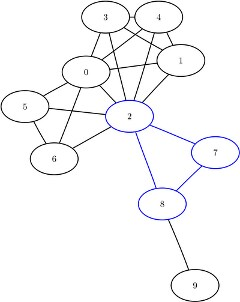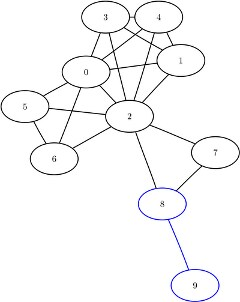### Clique

A clique of a graph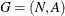is an induced subgraph that is a complete graph. Every node in a clique is connected to every other node in that clique. A maximal clique is a clique that is not a subset of the nodes of any larger clique. That is, it is a set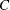of nodes such that every pair of nodes inis connected by a link and every node not inis missing a link to at least one node in. The number of maximal cliques in a given graph can be very large and can grow exponentially with every node added. Finding cliques in graphs has applications in numerous industries including bioinformatics, social networks, electrical engineering, and chemistry.

You can find the maximal cliques of an input graph by invoking the CLIQUE statement. The options for this statement are described in the section CLIQUE Statement. This algorithm works only with undirected graphs.

The results for the clique algorithm are written to the output data set that is specified in the OUT= option in the CLIQUE statement. Each node of each clique is listed in the output data set along with the variable clique to identify the clique to which it belongs. A node can appear multiple times in this data set if it belongs to multiple cliques.

The algorithm used by PROC OPTNET to compute maximal cliques is a variant of the Bron-Kerbosch algorithm (Bron and Kerbosch, 1973; Harley, 2003). Enumerating all maximal cliques is NP-hard, so this algorithm typically does not scale to very large graphs.

#### Maximal Cliques of a Simple Undirected Graph

This section illustrates the use of the clique algorithm on the simple undirected graph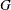shown in Figure 2.15.

Figure 2.15: A Simple Undirected Graph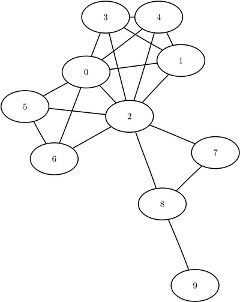The undirected graphcan be represented by the links data set LinkSetIn as follows:

data LinkSetIn;
input from to @@;
datalines;
0 1  0 2  0 3  0 4  0 5
0 6  1 2  1 3  1 4  2 3
2 4  2 5  2 6  2 7  2 8
3 4  5 6  7 8  8 9
;


The following statements calculate the maximal cliques, output the results in the data set Cliques, and use the SQL procedure as a convenient way to create a table CliqueSizes of clique sizes:

proc optnet
clique
out     = Cliques;
run;

proc sql;
create table CliqueSizes as
select clique, count(*) as size
from Cliques
group by clique
order by size desc;
quit;


The data set Cliques now contains the maximal cliques of the input graph; it is shown in Figure 2.16.

Figure 2.16: Maximal Cliques of a Simple Undirected Graph

clique node
1 0
1 2
1 1
1 3
1 4
2 0
2 2
2 5
2 6
3 2
3 8
3 7
4 8
4 9

In addition, the data set CliqueSizes contains the number of nodes in each clique; it is shown in Figure 2.17.

Figure 2.17: Sizes of Maximal Cliques of a Simple Undirected Graph

clique size
1 5
2 4
3 3
4 2

The maximal cliques are shown graphically in Figure 2.18 and Figure 2.19.

Figure 2.18: Maximal Cliques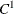and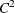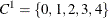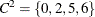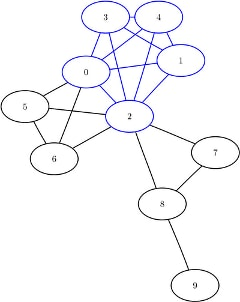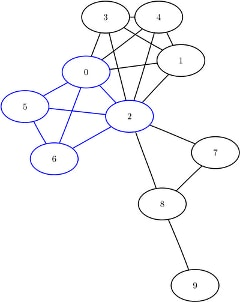Figure 2.19: Maximal Cliquesand